# TIME Function

Create a time with individual hour, minute and second components

## What is the TIME Function?

The TIME function is categorized under Excel DATE/TIME functions. TIME helps us to create a time with individual hour, minute, and second components.

While doing financial analysis, we might wish to put a time stamp on a report. In such scenarios, TIME can be used to convert a text string into a decimal format that represents the time. The function can also be used to merge individual values into a single time value.

### Formula

=TIME(hour, minute, second)

The TIME function uses the following arguments:

1. Hour (required argument) – This can be from 0 (zero) to 32767 representing the hour. If a value is more than 23 then it shall be divided by 24 and Excel shall treat the remainder as the hour value.
2. Minute (required argument) – Can be any number from 0 (zero) to 32767, representing the minutes. If a value is more than 59 then it is converted to hours and minutes.
3. Second (required argument) – It can be from 0 (zero) to 32767, representing the seconds. If a value is more than 59 then it is converted to hours, minutes and seconds.

The decimal number that is returned by the TIME function is a value between 0 (zero) to 0.99988426, representing the times from 0:00:00 (12:00:00 AM) to 23:59:59 (11:59:59 P.M.).

### How to use the TIME Function in Excel?

As a worksheet function, TIME can be entered as part of a formula in a cell of a worksheet.

To understand the uses of the TIME function, let’s consider a few examples:

#### Example 1

Suppose we extracted data from a PDF file and got the following data: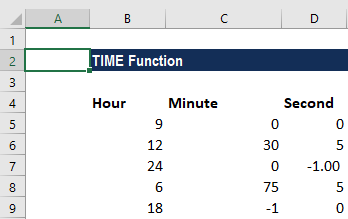Now, if we wish to put the time in the cell itself, then we use the TIME function. The formula to be used would be =TIME(B5,C5,D5) that TIME(hour, minutes, seconds).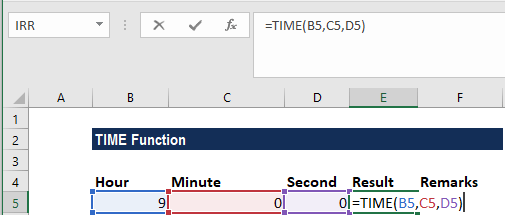We get the results below: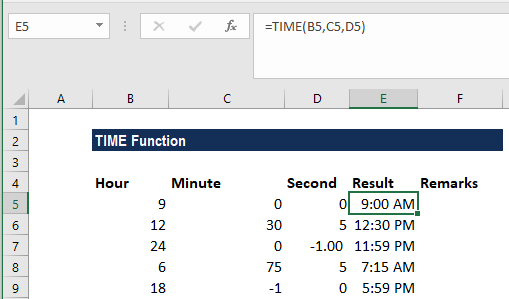If the end result is a negative number, TIME will return a #NUM! error. In row 4, the hours are 24, which is greater than 23. Any value greater than 23 will be divided by 24, with the remainder treated as the hour value. So, 24 would be 00 and we got -1 as seconds, which is negative, so it got subtracted to give 11:59. Remember, if the supplied second argument is negative or is greater than 59, the time extends back or forward into the previous or following minute.

If the given minute argument is negative or is greater than 59, the time extends back or forward into the previous or following hour. So, in row 6, we get the result as 5:59 pm.

#### Example 2

If we wish to add time to a given number of hours, we can add hours, divided by 24, or use the TIME function. Suppose we are given the following data: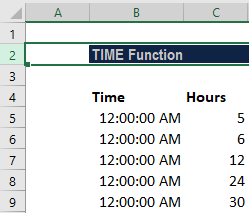We can use the TIME function to add the number of hours to the given time. The function saves us from the need to remember the formula for converting decimal hours to an Excel time. The formula to be used is =A2+TIME(B2,0,0).

We get the results below: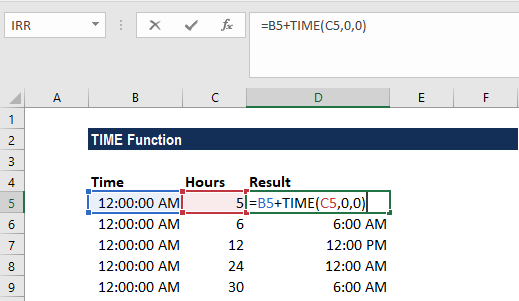Remember that the TIME function will “roll over” back to zero when values exceed 24 hours, as seen in rows 5 and 6 above.

If we wish to subtract hours from a given time, we need to use the formula =MOD(A2-TIME(B2,0,0),1).

The MOD function will take care of the negative numbers problem. MOD will “flip” negative values to the required positive values.

### Things to remember about the TIME function

1. TIME was introduced in MS Excel 2000 and is available in all later versions.
2. #NUM! error – Occurs if the given hour arguments evaluate to a negative time, e.g., if the given hours are less than 0.
3. #VALUE! error – Occurs when any of the given arguments is non-numeric.

Thanks for reading CFI’s guide to important Excel functions! By taking the time to learn and master these functions, you’ll significantly speed up your financial analysis. To learn more, check out these additional CFI resources:

• Excel Functions for Finance